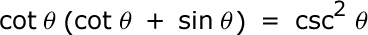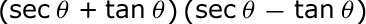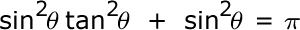# List of the Basic Trig Identities - Quiz & Worksheet

Instructions:

question 1 of 3

### What is the value of cos(θ) given that the equation below is true?Create Your Account To Take This Quiz

As a member, you'll also get unlimited access to over 84,000 lessons in math, English, science, history, and more. Plus, get practice tests, quizzes, and personalized coaching to help you succeed.

Try it risk-free for 30 days. Cancel anytime

### 1. Simplify the following expression.### 2. What's the value of sec2 (θ) given that the equation below is true?Create your account to access this entire worksheet
Quizzes, practice exams & worksheets
Certificate of Completion
Create an account to get started

The basic trigonometric identities, otherwise referred to as Pythagorean Identities, can help you group things together in very specific ways that will simplify them. This quiz and worksheet combo will allow you to test your understanding of these identities. The practice questions test your understanding of these identities and how to use them to simplify trigonometry problems.

## Quiz & Worksheet Goals

In these assessments, you'll be tested on:

• The trigonometric identities discussed in the lesson
• Your ability to use these identities to simplify trigonometry problems

## Skills Practiced

This quiz and worksheet will test your skills in the following areas:

• Reading comprehension - ensure that you draw the most important information on the Pythagorean Identities from the related lesson
• Problem solving - use your acquired knowledge to solve practice problems involving simplification of trigonometric problems using the identities discussed in this lesson
• Distinguishing differences - compare and contrast topics from the lesson, such as the separate identities discussed and what they allow you to calculate
• Information recall - access the knowledge you've gained regarding these identities and use that knowledge to answer questions about them

To learn more, review the accompanying lesson titled List of the Basic Trig Identities. This lesson covers the following objectives:

• Define the identities discussed as the Pythagorean Identities
• Equate the Pythagorean Theorem to the identities discussed
• Understand how to use these identities to simplify complicated trigonometric problems
• Demonstrate your ability to use these identities to simplify trigonometric problems
Final ExamMath 103: Precalculus
Status: Not Started
Chapter ExamTrigonometry
Status: Not Started

Support